# How to use the INDIRECT function in Google SheetsThe INDIRECT function in Google Sheets takes in the cell address in the form of text and returns a cell reference. It works in the opposite way to the ADDRESS function, which returns an address in text format.

The difference between the INDIRECT function and a typical direct function is that a typical function directly references a cell (or range of cells) within the formula. In contrast, the INDIRECT function uses the cell address (in the form of a text string) to find the relevant cell.

Using a text string to reference a cell provides several advantages that are outlined below.

### Syntax

INDIRECT(cell_reference_as_string, [is_A1_notation])

cell_reference_as_string – is the text form of a cell address. Notice that it is not the cell reference itself. Because it is in the form of a text string, it should be enclosed in double quotation marks unless it is referencing a cell that holds the text string (see the examples below).

is_a1_notation – this is an optional input that tells the function what type of notation the cell address is in. There are two kinds of representations for a cell address. A1 notation specifies the cell by the column letter (A, B, C, etc.) and the row number (1, 2, 3, etc.), identifying cells in the form of A1, B3, F14, and so on. Another way of representing a cell is R1C1, which gives the row number (R1) and the column number (C1). In this format, cell A1 would be R1C1, and cell B3 would be R3C2 (because B3 is the third row in the second column). TRUE is the value by default, and you get A1 notation in return. Otherwise, if you need R1C1 notation, you need to specify FALSE.

### How to use the INDIRECT function

The easiest way to understand this concept is to see it in use, so I’ve provided some examples below, each using a different combination.

As you can see, the first parameter can be a direct string enclosed in double quotes (rows 2 and 3), a cell reference that holds the address string (row # 7), or even a concatenated string (rows 4 and 5).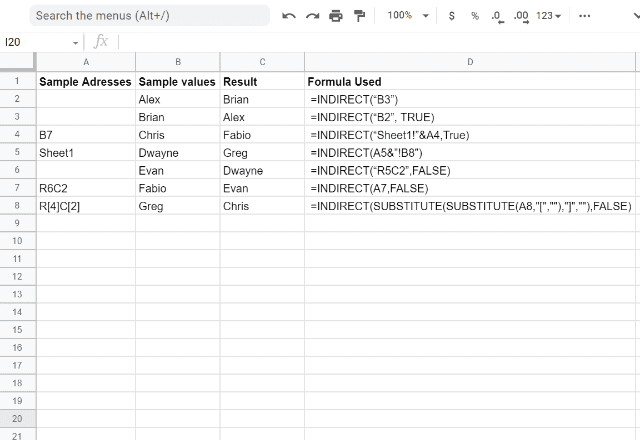To help you understand the function better, I’ll go through the list of the different variations of the formula and the results that each one generates.

#### Example 1

In the first formula =INDIRECT(“B3”), I simply specify that I want the result of the formula to be whatever is in cell B3, which is Brian.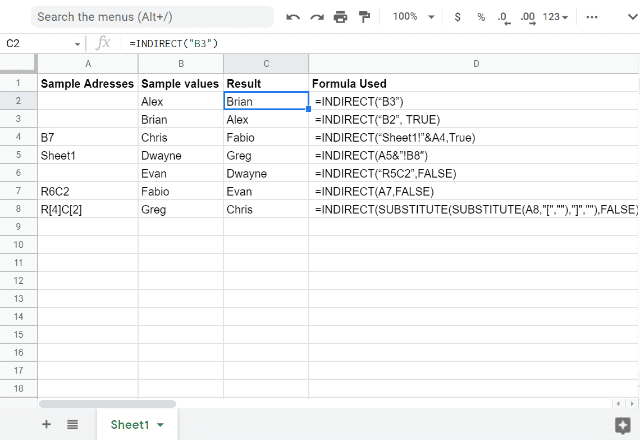#### Example 2

In the second formula =INDIRECT(“B2”, TRUE), I do the same thing, but this time I ask for the result to be from cell B2, which is Alex.

I also indicate TRUE, which means I want A1 notation.

Note that you don’t need to specify this. The function assumes that you are using A1 notation unless you indicate otherwise (by using FALSE which indicates R1C1 notation).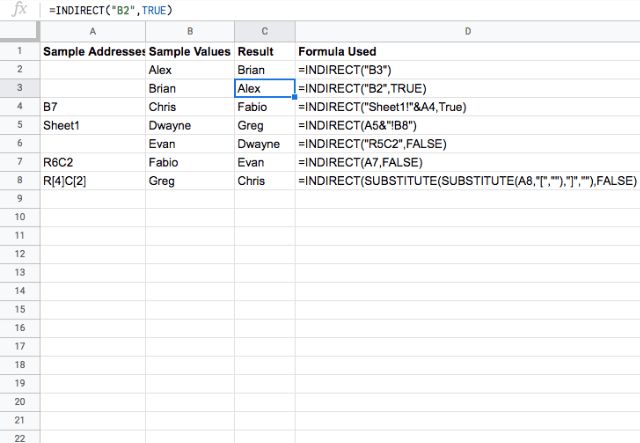#### Example 3

In the third formula =INDIRECT(“Sheet1!”&A4,True) I concatenate a text string that will tell the formula which cell I want to reference.

To do this, you start by saying “Sheet1!”, followed by &A4, which tells the formula to look at cell A4 on Sheet 1.

The text in cell A4 is “B7”, which tells the formula to give the output from cell B7, which is Fabio.

In this formula, I also indicate TRUE for the A1 notation, which is again optional.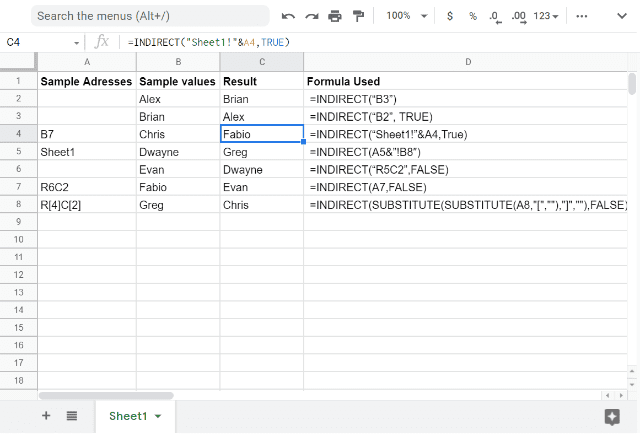#### Example 4

The fourth formula =INDIRECT(A5&”!B8″) does the same thing but in a different way.

It spells out “Sheet1!B8” by referencing cell A5 (which contains “Sheet1”) and adding the “!B8”.

As a result, the indirect formula looks at cell B8 and returns Greg.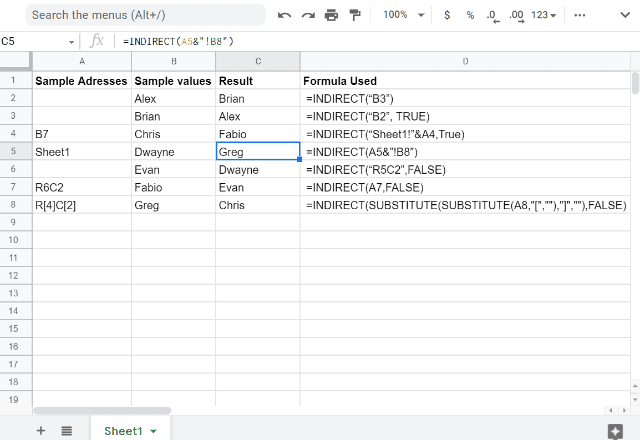#### Example 5

The fifth formula =INDIRECT(“R5C2”,FALSE) uses R1C1 format to identify cell B5 by saying R5C2 (the fifth row in the second column).

In this case, I must let the formula know that I’m using R1C1 notation by adding FALSE at the end of the formula.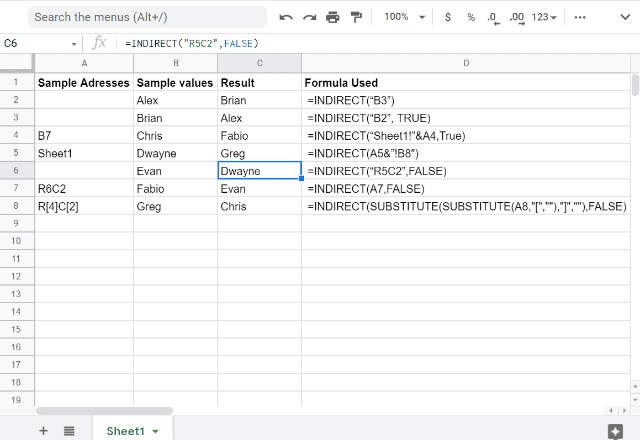#### Example 6

The sixth formula =INDIRECT(A7,FALSE) references another cell, A7, which points the formula to cell B6 in R1C1 format, so again I add FALSE.

Notice that in this example A7 is not in quotation marks because it is not a text string but a reference to the A7 cell that holds the text string (R6C2).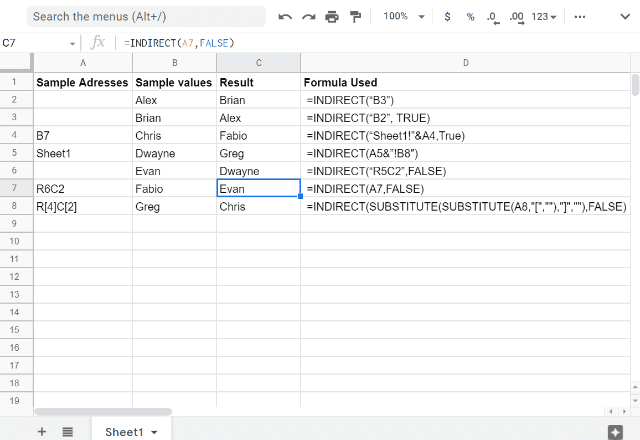The last formula =INDIRECT(SUBSTITUTE(SUBSTITUTE(A8,”[“,””),”]”,””),FALSE) uses the SUBSTITUTE formula to change the RC cell address format to R1C1, since this is the notation that the formula can handle.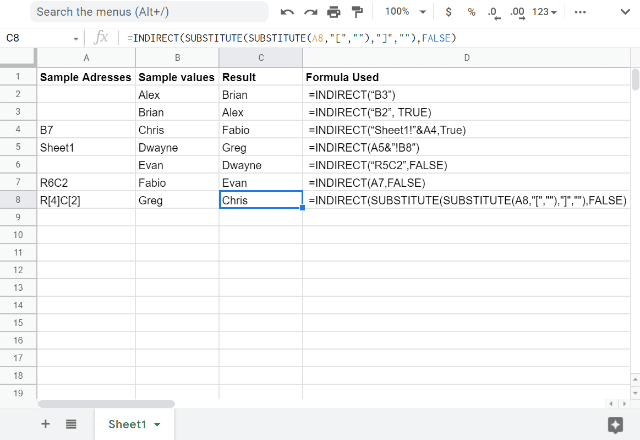### Maintaining a cell range in a formula

One of the benefits of using the INDIRECT function is that by specifying the cell(s) you want the formula to reference, those cells stay the same even if you add new rows or columns to the sheet.

The example below shows the difference between using a regular SUM formula, and using the SUM formula combined with an INDIRECT formula, =SUM(INDIRECT(“B2:B6”))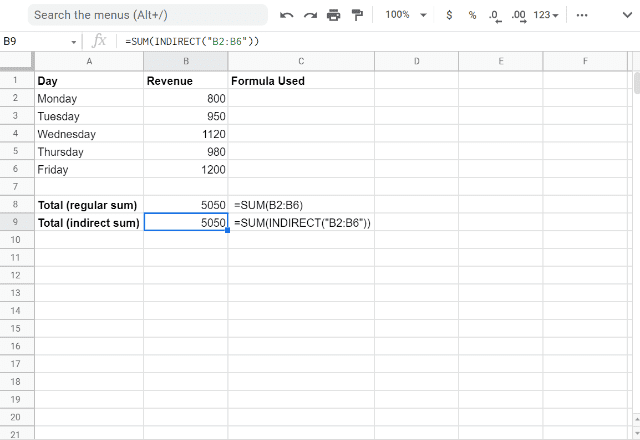Here, you can see that to add up the revenue for Monday to Friday, SUM can be used either with or without the INDIRECT function.

Sum function references the cells B2:B6 directly while using the INDIRECT formula references the cells as a text string “B2:B6”.

The difference comes into play when you make changes to the sheet such as by adding another row.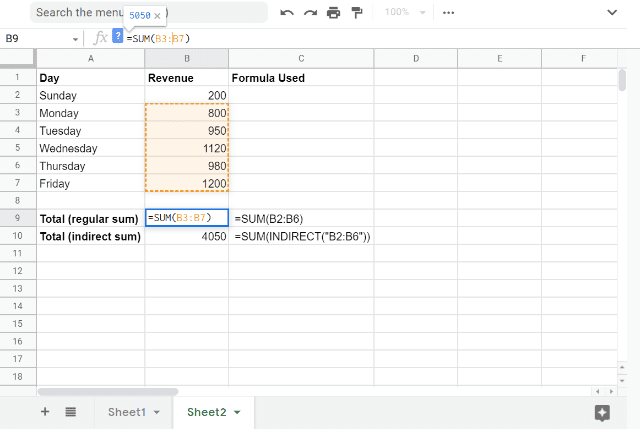In the screenshot above, you can see that an extra row has been inserted above Monday in order to add revenue from Sunday. When this happens, the SUM function automatically changes its cells to B3:B7.

The formula that used the INDIRECT function, however, maintained the original range of B2:B6.

In some situations, the fact that SUM (and other formulas) can change its range based on alterations to the rows and columns in a sheet can be helpful. On the other hand, this might be frustrating when you want to keep the range the same.

In these cases, the INDIRECT function is a useful way of achieving this.

### Using INDIRECT with Named Ranges

Named ranges can be created to refer to a set group of cells. The INDIRECT formula can use a named range for a variety of calculations.

In the example below, I’ve created a named range for cells B3:B7 called Monday_to_Friday.

This can be done by selecting the cells and clicking Data > Named Ranges.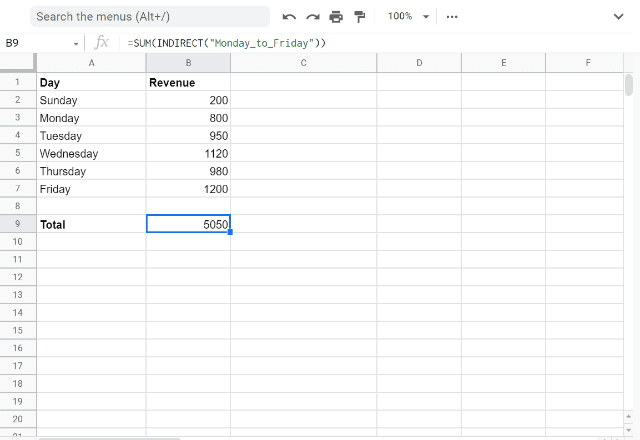Once the named range is created, it can be referenced using the INDIRECT function.

In the example above, I use =SUM(INDIRECT(“Monday_to_Friday”)) to call up the Monday_to_Friday range and then output the sum of this range.

### How to use INDIRECT to reference other sheets

Another use of the INDIRECT function is referencing data in other sheets.

In the simple example below, you can see how the function can be used to pull data from other sheets.

I’ve created a second tab called Sheet2, and on cell B4 of that sheet, I’ve entered “Data from Sheet 2”.

Then on Sheet 1, I can use the INDIRECT formula to refer to that cell and output the data from that cell by saying =INDIRECT(“Sheet2!”&”B4”)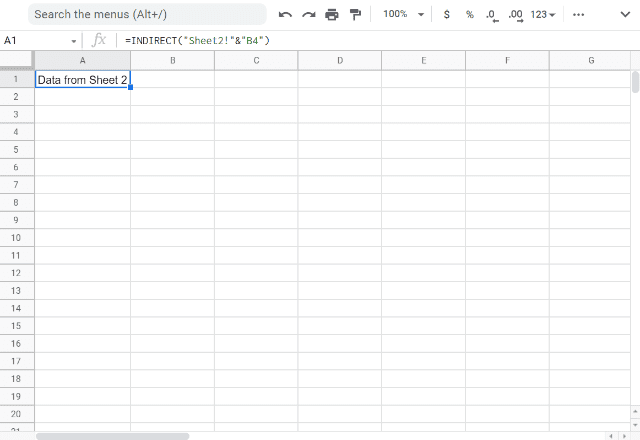This can be useful when you have a sheet with multiple tabs that you are pulling information from. Instead of including Sheet2 in the formula, you can write “Sheet2” in a cell, then have the INDIRECT formula refer to that cell.

If you then want to change it to get data from another tab, instead of changing the formula you can simply change the cell to say “Sheet3” instead of Sheet2, for example.

Likewise, you can do the same with the specific cell that you’re referencing on the sheet (B4 in this example).

The INDIRECT function can also be used to use conditional formatting with multiple tabs.

### How to use the INDIRECT function with MATCH

The INDIRECT function can be combined with the MATCH function to return a dynamic range of cells.

In the following example, I combine the INDIRECT and MATCH functions to give me the revenues for the days that I specify, using the formula =INDIRECT(“B” & MATCH(B10,A:A,FALSE) & “:B” & MATCH(B11,A:A,FALSE), TRUE)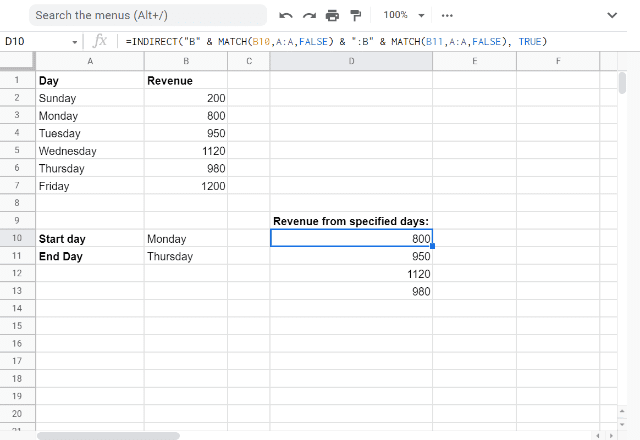As you can see from the screenshot, I’ve entered Monday and Thursday as the start and end days that I want to view revenue for.

The MATCH formula finds those days in my data range and identifies the rows that they’re in. These rows are put into the INDIRECT formula as the rows between which I want to return the data. The result is that the formula gives the revenues from Monday to Thursday.

By setting it up this way, I can get data from different ranges of days just by changing the start and end day cells.

I can also combine this formula with other functions, such as SUM, to give me the overall revenue from the range of days I specify.

I’ve done this in the example below, by typing =SUM(INDIRECT(“R” & MATCH(B10,A:A,FALSE) & “C2” & “:R” & MATCH(B11,A:A,FALSE) & “C2”, false))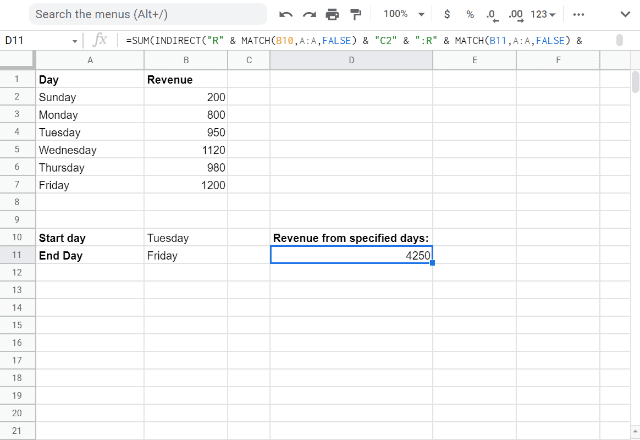As you can see, I’ve used R1C1 notation in this example instead of the A1 notation of the previous example.

However, the logic is the same: use the MATCH function to identify the data you want to get and use the INDIRECT function to call up that data.

### Using INDIRECT formula with tabs

The INDIRECT formula can be used to consolidate information from multiple tabs in a spreadsheet.

In the following example, I have a spreadsheet with sales data for the year. Each month is a separate tab, which has weekly sales data and a total sales value for the month, as shown in the following screenshot.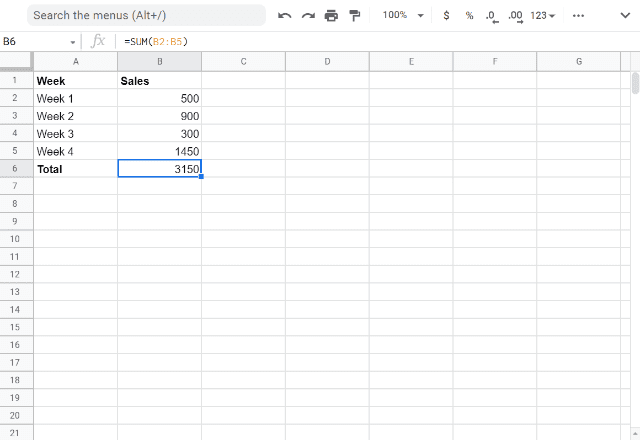In the Summary tab, I want to bring in all the monthly sales totals.

Instead of copying and pasting each value, I can use the INDIRECT formula to access data in each tab using the following formula: =INDIRECT(A2&”!B6″)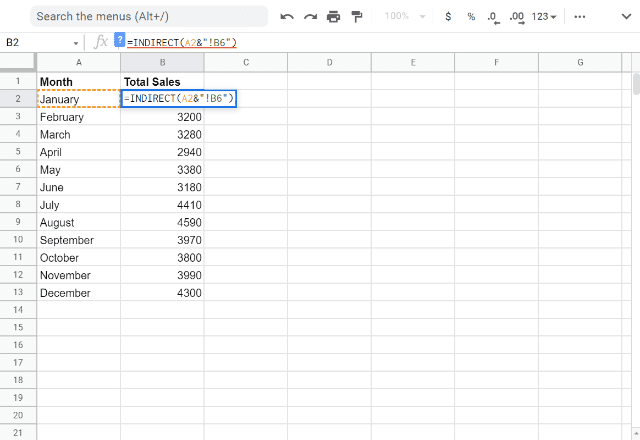As you can see, this formula tells the indirect function to look in each tab and return the total sales for that month, which is in cell B6 of each tab.

By doing this, you can quickly consolidate data across multiple tabs. The best part is that when the numbers change in any of the tabs, that change is reflected in the summary tab automatically!

### Errors – INDIRECT formula

Will the INDIRECT formula result in errors? Yes, of course!

The following screenshot illustrates the outcome when you try to input A1 style address notation for cell_reference_as_string and FALSE for the is_A1_notation parameter.

Doing this will obviously leave the INDIRECT formula looking for R1C1 style notation, and it doesn’t find one. Hence the error!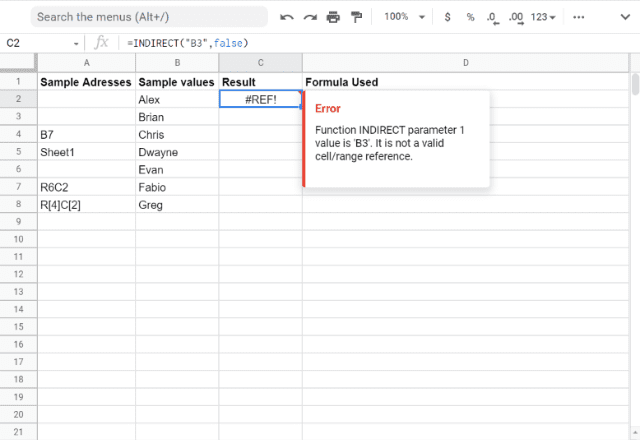The vice versa is also true, as indicated in the snapshot below.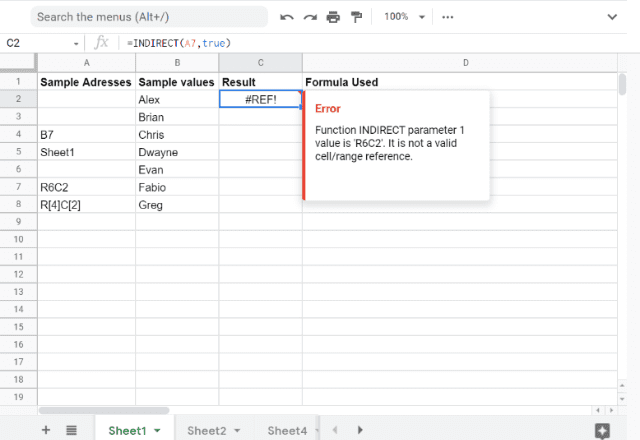Now the worst-case scenario.

You try to input a text form that is neither A1 representation nor the R1C1 representation, but just some dummy text. And, of course, it should throw up an error!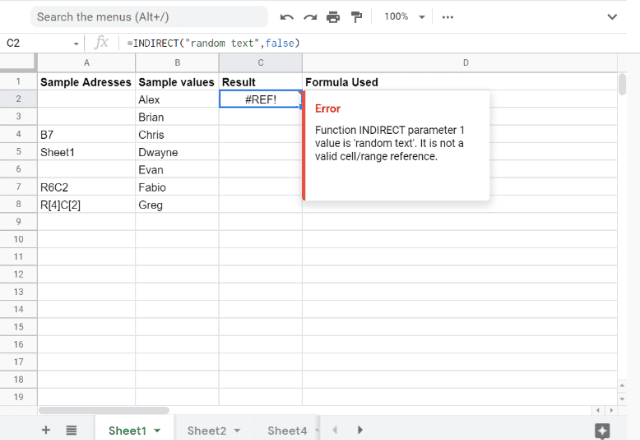Thanks for reading — that’s Sheetgo’s in-depth guide on how to use the INDIRECT function in Google Sheets. Check out our other articles for more spreadsheet tips!

### Did you like this post?

Share it with your friends and colleagues via the social media buttons on the left.

Editor’s note: This is a revised version of a previous post that has been updated for accuracy and comprehensiveness.